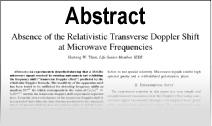Enter the content which will be displayed in sticky bar

# AbstractGravitons, the Speed of Gravity, and the Generalized Newton Gravitational Law

Year: 2013 Pages: 6
Keywords: speed of gravity, aether, dark matter, stability of the planetary orbits, Newton third law of action and reaction, gravitons, generalized Newton gravitational law, aberration of gravity, barycenter

In many publications and web forum discussions the claims are constantly being made that in order for the orbits of planets around the Sun to be stable the gravity must propagate at much higher speeds than the speed of light c. In this paper it is shown on a simple and extreme example of the two stars orbiting around each other in a circular orbit that this is not the case and that the assumption about the necessity for the large speed of gravity is unfounded. The explanation is based on the recognition that the Newton gravitational force has the two components that are not necessarily collinear. This new fundamental finding is supported by modeling the field by gravitons that mediate the force of the field. This model finally leads to the generalization of the Newton gravitational law that correctly accounts for the finite speed of gravity. From this result it is also found that the gravitational aberration angle is identical with the aberration angle of light, but is aiming in the opposite direction, lagging behind the source of the gravitational attraction.